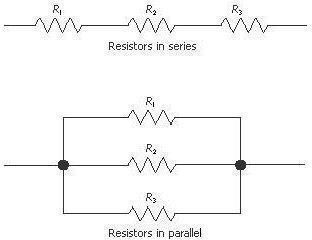# Learn About Series and Parallel Resistors – Electrical Engineering Basics

## Introduction

What do you do when you have a very big source of water say like a river or canal and want to give water to a small field? Well if you simply lay the field open to the river or the canal it would simply get submerged and rendered useless for whatever purposes it was intended such as irrigation. So the viable and wise option is to connect the field via a restricted channel which offers resistance to the flow of water so that only that much water enters the field as can be safely handled by it without getting damaged.

You might be wondering what this has to do with electrical or electronics technology but here is the catch. If you have a huge power source and want to power a smaller component you need to divide the current or the voltage as required. This is done by using appropriate electronic circuitry of which resistors are an integral part. So let us see in the next section how resistors and their various combinations are used to alter voltage and current in circuits

## The Series Arrangement

Resistors are said to be connected in series arrangement when they are joined end to end with one another so that the current flows out of the first resistor and enters the second one, and so on. Let us suppose there are 3 resistors (this is just a random number, the rules described here apply to more or less numbers as well) having values R1, R2 and R3 ohms respectively.

Now if these resistors are connected in series then the total resistance of their combination is the sum total of their individual resistances which can be written mathematically as follows

R = R1 +R2 + R3

Where R is the total resistance

Other characteristics of this arrangement are that the current remains the same in all resistors obviously because it has only got a single path to flow but the potential difference or the voltage across each resistor varies in proportion to the size of the resistor

If you remember the equation V = I * R

Then if the current remains the same (as in case of series arrangement), the voltage varies proportionately to resistance. This means that the bigger resistor would have a greater drop across it and vice versa.

## The Parallel Arrangement

Sometimes it must be so required that the voltage required might be the same across different sections but current requirements are different. Hence in such a case the resistors are not joined end to end but in parallel so that one end of all resistors is joined together at one side, while the other ends join together on the other side and this obviously is known as the parallel arrangement.

In this case the current has now three different paths to flow so it gets divided in proportion to the ease with which it can flow into each resistor. This means that the greater resistance would have smaller current flowing across it and vice versa. There is another difference here that the voltage across all resistors is the same unlike the parallel arrangement described in the previous section.

The total resistance R in such a case is given by

1/R = 1/R1 + 1/R2 + 1/R3

You can view both types of arrangements in the figure below

## Series & Parallel Resistors